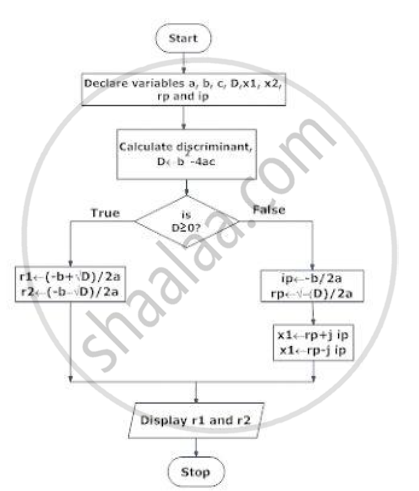# Draw the Flowchart for Finding the Roots of Quadratic Equation. Write Program for Same. - Structured Programming Approach

Draw the flowchart for finding the roots of quadratic equation. Write program for same.

#### Solution}
else if (discriminant == 0)
{
r1 = r2 = -b/(2*a);
printf("r1 = r2 = %.2lf;", r1);
}
else
{
rp = -b/(2*a);
ip = sqrt(-discriminant)/(2*a);
printf("r1 = %.2lf+%.2lfi and r2 = %.2f-%.2fi", rp, ip, rp, ip);
}
return 0;
}

 Output:Enter coefficients a, b and c: 2.345.6Roots are: -0.87+1.30i and -0.87-1.30i
Concept: Three Construct of Algorithm and Flowchart
Is there an error in this question or solution?
2018-2019 (December) CBCGS

Share